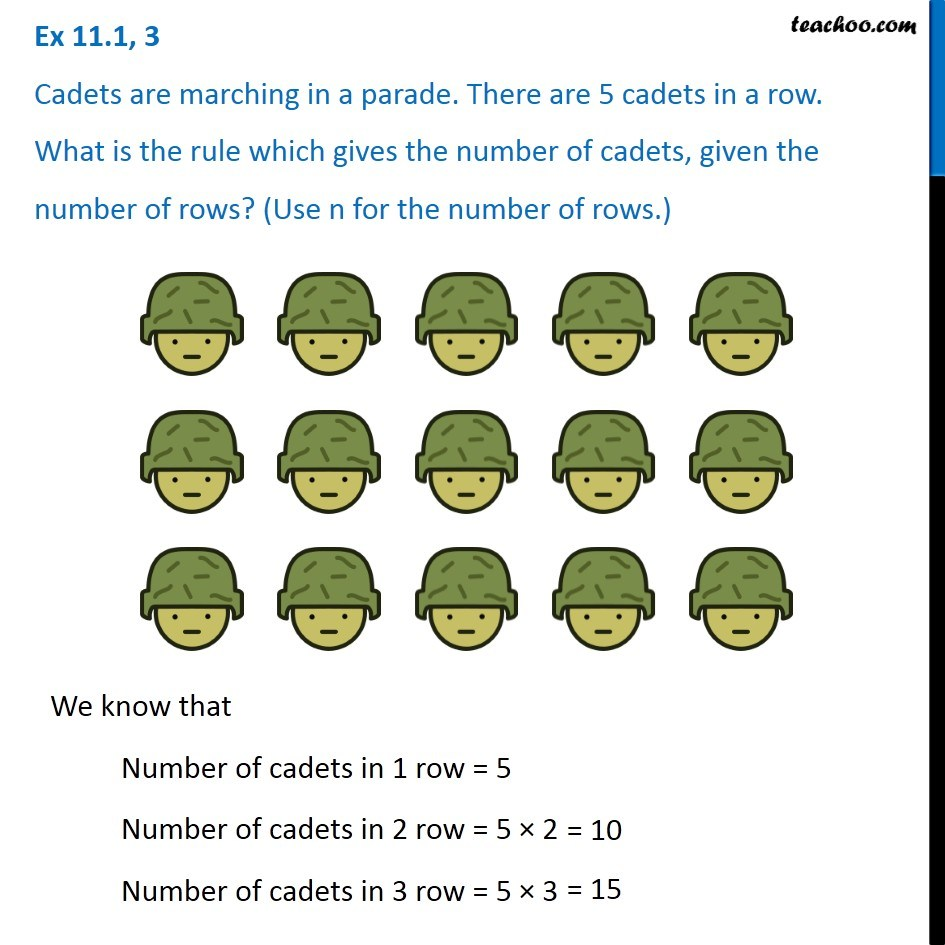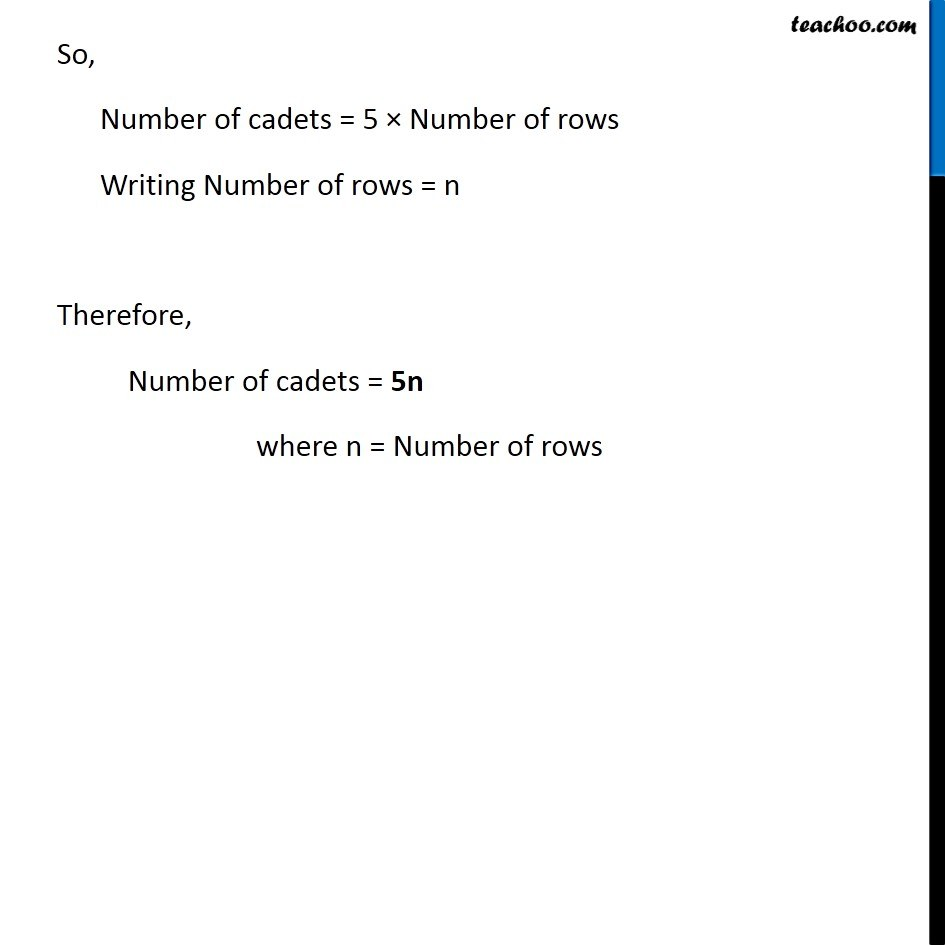Ex 11.1

Chapter 11 Class 6 Algebra
Serial order wiseLearn in your speed, with individual attention - Teachoo Maths 1-on-1 Class

### Transcript

Ex 11.1, 3 Cadets are marching in a parade. There are 5 cadets in a row. What is the rule which gives the number of cadets, given the number of rows? (Use n for the number of rows.) We know that Number of cadets in 1 row = 5 Number of cadets in 2 row = 5 × 2 Number of cadets in 3 row = 5 × 3 So, Number of cadets = 5 × Number of rows Writing Number of rows = n Therefore, Number of cadets = 5n where n = Number of rows